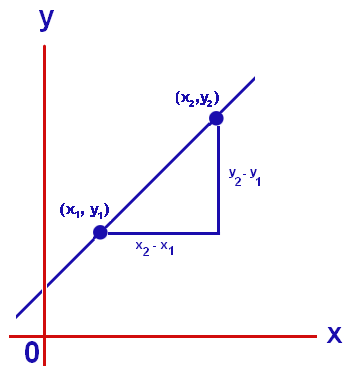# Rate of Change Formula

The dictionary meaning of slope is a gradient, pitch or incline. This formula is used to measure the steepness of a straight line. The higher the slope, the steeper it gets. That slope is knows as the rate of change. The slope of the line that connects the points of the line. The rate of change to the coordinates of y to coordinates of x in slope can found out if the coordinates of any two points is given.The formula for rate of change is:

$\large Rate\;of\;Change= \frac{y_{2}-y_{1}}{x_{2}-x_{1}}$

### Solved example

Question: By how much has the value of y changed between the two points (-4, -7) and (-2, -6)?

Solution:

Here,

$$\begin{array}{l}x_{1}, y_{1}\end{array}$$
= (-4, -7)
$$\begin{array}{l}x_{2}, y_{2}\end{array}$$
=
(-2, -6)

With the formula we can evaluate the rate of change:

$$\begin{array}{l}Rate\;of\;Change= \frac{y_{2}-y_{1}}{x_{2}-x_{1}}\end{array}$$

$$\begin{array}{l}Rate\;of\;Change= \frac{-6-(-7)}{-2-(-4)}=\frac{1}{2}\end{array}$$